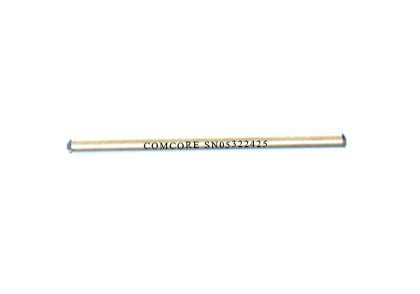### Current Position：Home / Products

 1x2(2x2) Ultra-Low PDL Broadband SplitterProduct Features Product Applications Ultra-Low PDL Optical Communication System Low Insertion Loss Optical Testing System High Directivity Optical Fiber Sensor Stable and Reliable Optical Power Distributor
 Specifications Splitting Ratio:50:50 Parameter Unit Premium A grade Port Configuration 1x2 or 2x2 Bandwidth nm ± 40 Insertion Loss Max dB 3.4 3.6 Excess Loss Typ dB 0.07 0.1 Uniformity Max dB 0.6 1.0 PDL Max dB 0.05 0.07 Return Loss* Min dB 55 50 Operating Power Max W 5 Operating Temperature °C -40 to +85 Storage Temperature °C -50 to +85 Package Type mm S6=Ø3x54: for bare fiber S8=Ø3x70: for 0.9mm loose tube M1=9x16x90: for 0.9mm loose tube or 2mm cable or 3mm cable

*Test at central wavelength only. There would be an unused termination port around 20cm for 1x2 version.

 Splitting Ratio & Insertion Loss Conversion Table Splitting Ratio Maximum Insertion Loss (dB) Premium A grade Output Port 1 Output Port 2 Output Port 1 Output Port 2 50:50 3.4 3.4 3.6 3.6 60:40 2.5 4.4 2.8 4.8 70:30 1.8 5.6 2.0 6.1 80:20 1.2 7.5 1.3 8.0 90:10 0.6 10.8 0.8 12
 Ordering Information L B S Wavelength 1=1625nm 2=1590nm 3=1570nm 4=1550nm 5=1480nm 6=1475nm 7=1310nm P=2000nm S=Specify Structure 1=1x2 2=2x2 Splitting Ratio 90=90:10 80=80:20 70=70:30 60=60:40 50=50:50 ...... Grade P=Premium A=A grade Package 5=S6 7=S8 D=M1 Fiber Type 1=G652 or Equivalent H=SM 1950 Pigtail S=250μm bare fiber M=0.9mm loose tube L=3mm cable R=2mm cable Fiber Length 0=0.5m 1=0.75m 2=1.0m 3=1.5m 4=2.0m S=Specify Connector 0=None 1=FC/PC 2=FC/SPC 3=FC/APC 4=SC/SPC 5=SC/APC 6=ST 7=FC/UPC 8=SC/UPC 9=MU A=LC/PC B=SC/PC C=LC/UPC D=LC/APC

Note:1. Central wavelength can be customized for different applications.
2. All specifications are before connectors and are subject to change without notice.